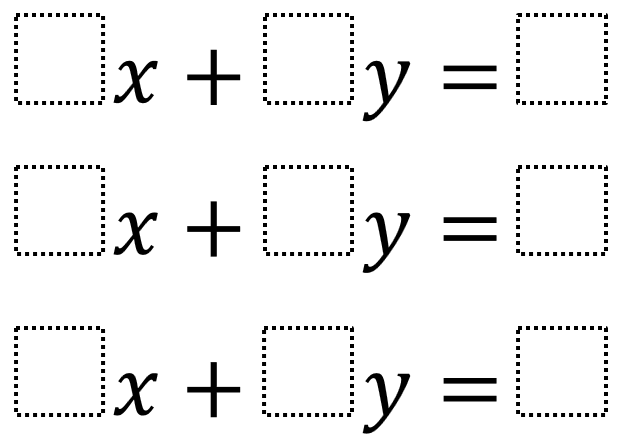# Integer Y-Intercepts

Directions: Using the digits 1 to 9 at most one time each, place a digit in each box to create three different linear equations whose y-intercepts have integer values.### Hint

What is the x-coordinate of a y-intercept? What will the equation look like when it is written in slope-intercept form?